## Fermat's Last Theorem

A theorem first proposed by Fermatin the form of a note scribbled in the margin of his copy of the ancient Greek text Arithmetica by Diophantus.The scribbled note was discovered posthumously, and the original is now lost. However, a copy was preserved in a book published by Fermat's son. In the note, Fermat claimed to have discovered a proof that the Diophantine Equationhas no Integer solutions for.

The full text of Fermat's statement, written in Latin, reads Cubum autem in duos cubos, aut quadratoquadratum in duos quadratoquadratos & generaliter nullam in infinitum ultra quadratum potestatem in duos eiusdem nominis fas est diuidere cuius rei demonstrationem mirabilem sane detexi. Hanc marginis exiguitas non caperet.'' In translation, It is impossible for a cube to be the sum of two cubes, a fourth power to be the sum of two fourth powers, or in general for any number that is a power greater than the second to be the sum of two like powers. I have discovered a truly marvelous demonstration of this proposition that this margin is too narrow to contain.''

As a result of Fermat's marginal note, the proposition that the Diophantine Equation(1)

where,,, andare Integers, has no Nonzero solutions forhas come to be known as Fermat's Last Theorem. It was called a Theorem'' on the strength of Fermat's statement, despite the fact that no other mathematician was able to prove it for hundreds of years.

Note that the restrictionis obviously necessary since there are a number of elementary formulas for generating an infinite number of Pythagorean Triplessatisfying the equation for,(2)

A first attempt to solve the equation can be made by attempting to factor the equation, giving(3)

Since the product is an exact Power,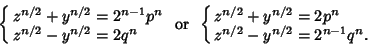(4)

Solving forandgives(5)

which give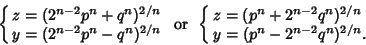(6)

However, since solutions to these equations in Rational Numbers are no easier to find than solutions to the original equation, this approach unfortunately does not provide any additional insight.

It is sufficient to prove Fermat's Last Theorem by considering Prime Powers only, since the arguments can otherwise be written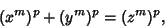(7)

so redefining the arguments gives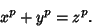(8)

The so-called first case'' of the theorem is for exponents which are Relatively Prime to,, and() and was considered by Wieferich. Sophie Germain proved the first case of Fermat's Last Theorem for any Odd Prime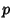when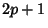is also a Prime. Legendresubsequently proved that ifis a Prime such that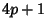,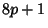,,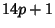, oris also a Prime, then the first case of Fermat's Last Theorem holds for. This established Fermat's Last Theorem for. In 1849, Kummerproved it for all Regular Primes and Composite Numbers of which they are factors (Vandiver 1929, Ball and Coxeter 1987).

Kummer'sattack led to the theory of Ideals, and Vandiver developed Vandiver's Criteria for deciding if a given Irregular Prime satisfies the theorem. Genocchi (1852) proved that the first case is true forifis not an Irregular Pair. In 1858, Kummer showed that the first case is true if eitheroris an Irregular Pair, which was subsequently extended to include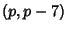andby Mirimanoff (1905). Wieferich (1909) proved that if the equation is solved in integers Relatively Prime to an Odd Prime, then(9)

(Ball and Coxeter 1987). Such numbers are called Wieferich Primes. Mirimanoff (1909) subsequently showed that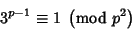(10)

must also hold for solutions Relatively Prime to an Odd Prime, which excludes the first two Wieferich Primes 1093 and 3511. Vandiver (1914) showed(11)

and Frobenius extended this to(12)

It has also been shown that ifwere a Prime of the form, then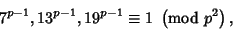(13)

which raised the smallest possiblein the first case'' to 253,747,889 by 1941 (Rosser 1941). Granville and Monagan (1988) showed if there exists a Primesatisfying Fermat's Last Theorem, then(14)

for, 7, 11, ..., 71. This establishes that the first case is true for all Prime exponents up to 714,591,416,091,398 (Vardi 1991).

The second case'' of Fermat's Last Theorem (for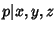) proved harder than the first case.

Eulerproved the general case of the theorem for, Fermat, Dirichletand Lagrange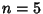. In 1832, Dirichletestablished the case. Thecase was proved by Lamé (1839), using the identity(15)
Although some errors were present in this proof, these were subsequently fixed by Lebesgue (1840). Much additional progress was made over the next 150 years, but no completely general result had been obtained. Buoyed by false confidence after his proof that Pi is Transcendental, the mathematician Lindemannproceeded to publish several proofs of Fermat's Last Theorem, all of them invalid (Bell 1937, pp. 464-465). A prize of 100,000 German marks (known as the Wolfskel Prize) was also offered for the first valid proof (Ball and Coxeter 1987, p. 72).

A recent false alarm for a general proof was raised by Y. Miyaoka (Cipra 1988) whose proof, however, turned out to be flawed. Other attempted proofs among both professional and amateur mathematicians are discussed by vos Savant (1993), although vos Savant erroneously claims that work on the problem by Wiles (discussed below) is invalid. By the time 1993 rolled around, the general case of Fermat's Last Theorem had been shown to be true for all exponents up to(Cipra 1993). However, given that a proof of Fermat's Last Theorem requires truth for all exponents, proof for any finite number of exponents does not constitute any significant progress towards a proof of the general theorem (although the fact that no counterexamples were found for this many cases is highly suggestive).

In 1993, a bombshell was dropped. In that year, the general theorem was partially proven by Andrew Wiles (Cipra 1993, Stewart 1993) by proving the Semistable case of the Taniyama-Shimura Conjecture. Unfortunately, several holes were discovered in the proof shortly thereafter when Wiles' approach via the Taniyama-Shimura Conjecture became hung up on properties of the Selmer Group using a tool called an Euler system.'' However, the difficulty was circumvented by Wiles and R. Taylor in late 1994 (Cipra 1994, 1995ab) and published in Taylor and Wiles (1995) and Wiles (1995). Wiles' proof succeeds by (1) replacing Elliptic Curves with Galois representations, (2) reducing the problem to a Class Number Formula, (3) proving that Formula, and (4) tying up loose ends that arise because the formalisms fail in the simplest degenerate cases (Cipra 1995a).

The proof of Fermat's Last Theorem marks the end of a mathematical era. Since virtually all of the tools which were eventually brought to bear on the problem had yet to be invented in the time of Fermat,it is interesting to speculate about whether he actually was in possession of an elementary proof of the theorem. Judging by the temerity with which the problem resisted attack for so long, Fermat's alleged proof seems likely to have been illusionary.

See also abc Conjecture, Bogomolov-Miyaoka-Yau Inequality, Mordell Conjecture, Pythagorean Triple, Ribet's Theorem, Selmer Group, Sophie Germain Prime, Szpiro's Conjecture, Taniyama-Shimura Conjecture, Vojta's Conjecture, Waring Formula

References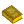Fermat's Last Theorem

Ball, W. W. R. and Coxeter, H. S. M. Mathematical Recreations and Essays, 13th ed. New York: Dover, pp. 69-73, 1987.

Beiler, A. H. The Stone Wall.'' Ch. 24 in Recreations in the Theory of Numbers: The Queen of Mathematics Entertains. New York: Dover, 1966.

Bell, E. T. Men of Mathematics. New York: Simon and Schuster, 1937.

Bell, E. T. The Last Problem. New York: Simon and Schuster, 1961.

Cipra, B. A. Fermat Theorem Proved.'' Science 239, 1373, 1988.

Cipra, B. A. Mathematics--Fermat's Last Theorem Finally Yields.'' Science 261, 32-33, 1993.

Cipra, B. A. Is the Fix in on Fermat's Last Theorem?'' Science 266, 725, 1994.

Cipra, B. A. Fermat's Theorem--At Last.'' What's Happening in the Mathematical Sciences, 1995-1996, Vol. 3. Providence, RI: Amer. Math. Soc., pp. 2-14, 1996.

Cipra, B. A. Princeton Mathematician Looks Back on Fermat Proof.'' Science 268, 1133-1134, 1995b.

Courant, R. and Robbins, H. Pythagorean Numbers and Fermat's Last Theorem.'' §2.3 in Supplement to Ch. 1 in What is Mathematics?: An Elementary Approach to Ideas and Methods, 2nd ed. Oxford, England: Oxford University Press, pp. 40-42, 1996.

Cox, D. A. Introduction to Fermat's Last Theorem.'' Amer. Math. Monthly 101, 3-14, 1994.

Dickson, L. E. Fermat's Last Theorem,, and the Congruence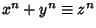(mod).'' Ch. 26 in History of the Theory of Numbers, Vol. 2: Diophantine Analysis. New York: Chelsea, pp. 731-776, 1952.

Edwards, H. M. Fermat's Last Theorem: A Genetic Introduction to Algebraic Number Theory. New York: Springer-Verlag, 1977.

Edwards, H. M. Fermat's Last Theorem.'' Sci. Amer. 239, 104-122, Oct. 1978.

Granville, A. Review of BBC's Horizon Program, Fermat's Last Theorem'.'' Not. Amer. Math. Soc. 44, 26-28, 1997.

Granville, A. and Monagan, M. B. The First Case of Fermat's Last Theorem is True for All Prime Exponents up to 714,591,416,091,389.'' Trans. Amer. Math. Soc. 306, 329-359, 1988.

Guy, R. K. The Fermat Problem.'' §D2 in Unsolved Problems in Number Theory, 2nd ed. New York: Springer-Verlag, pp. 144-146, 1994.

Hanson, A. Fermat Project.'' http://www.cica.indiana.edu/projects/Fermat/.

Kolata, G. Andrew Wiles: A Math Whiz Battles 350-Year-Old Puzzle.'' New York Times, June 29, 1993.

Lynch, J. Fermat's Last Theorem.'' BBC Horizon television documentary. http://www.bbc.co.uk/horizon/fermat.shtml.

Lynch, J. (Producer and Writer). The Proof.'' NOVA television episode. 52 mins. Broadcast by the U. S. Public Broadcasting System on Oct. 28, 1997.

Mirimanoff, D. Sur le dernier théorème de Fermat et le critérium de Wiefer.'' Enseignement Math. 11, 455-459, 1909.

Mordell, L. J. Fermat's Last Theorem. New York: Chelsea, 1956.

Murty, V. K. (Ed.). Fermat's Last Theorem: Proceedings of the Fields Institute for Research in Mathematical Sciences on Fermat's Last Theorem, Held 1993-1994 Toronto, Ontario, Canada. Providence, RI: Amer. Math. Soc., 1995.

Osserman, R. (Ed.). Fermat's Last Theorem. The Theorem and Its Proof: An Exploration of Issues and Ideas. 98 min. videotape and 56 pp. book. 1994.

Ribenboim, P. Lectures on Fermat's Last Theorem. New York: Springer-Verlag, 1979.

Ribet, K. A. and Hayes, B. Fermat's Last Theorem and Modern Arithmetic.'' Amer. Sci. 82, 144-156, March/April 1994.

Ribet, K. A. and Hayes, B. Correction to Fermat's Last Theorem and Modern Arithmetic.'' Amer. Sci. 82, 205, May/June 1994.

Rosser, B. On the First Case of Fermat's Last Theorem.'' Bull. Amer. Math. Soc. 45, 636-640, 1939.

Rosser, B. A New Lower Bound for the Exponent in the First Case of Fermat's Last Theorem.'' Bull. Amer. Math. Soc. 46, 299-304, 1940.

Rosser, B. An Additional Criterion for the First Case of Fermat's Last Theorem.'' Bull. Amer. Math. Soc. 47, 109-110, 1941.

Shanks, D. Solved and Unsolved Problems in Number Theory, 4th ed. New York: Chelsea, pp. 144-149, 1993.

Singh, S. Fermat's Enigma: The Quest to Solve the World's Greatest Mathematical Problem. New York: Walker & Co., 1997.

Stewart, I. Fermat's Last Time-Trip.'' Sci. Amer. 269, 112-115, 1993.

Taylor, R. and Wiles, A. Ring-Theoretic Properties of Certain Hecke Algebras.'' Ann. Math. 141, 553-572, 1995.

van der Poorten, A. Notes on Fermat's Last Theorem. New York: Wiley, 1996.

Vandiver, H. S. On Fermat's Last Theorem.'' Trans. Amer. Math. Soc. 31, 613-642, 1929.

Vandiver, H. S. Fermat's Last Theorem and Related Topics in Number Theory. Ann Arbor, MI: 1935.

Vandiver, H. S. Fermat's Last Theorem: Its History and the Nature of the Known Results Concerning It.'' Amer. Math. Monthly, 53, 555-578, 1946.

Vardi, I. Computational Recreations in Mathematica. Reading, MA: Addison-Wesley, pp. 59-61, 1991.

vos Savant, M. The World's Most Famous Math Problem. New York: St. Martin's Press, 1993.

Wieferich, A. Zum letzten Fermat'schen Theorem.'' J. reine angew. Math. 136, 293-302, 1909.

Wiles, A. `Modular Elliptic-Curves and Fermat's Last Theorem.'' Ann. Math. 141, 443-551, 1995.